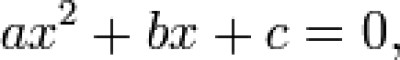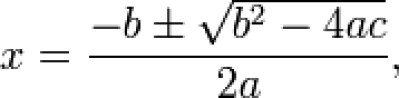List of public pages created with Protopage

# Trigonometry

## Plain sticky notes

### What are the 3 ways to work out trigonometry

Trigonometry is really very simple, all you have to is put the right numbers into an equation and put that into a calculator and Bob's your Uncle. There are 3 different things you use to work out trigonometry, Sine, Cosine and Tangent.

## Photos

This is the simple equation used to work out a trigonometry question using Cosine.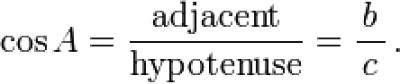These are the different sides of the triangle that you will need to work out the equation.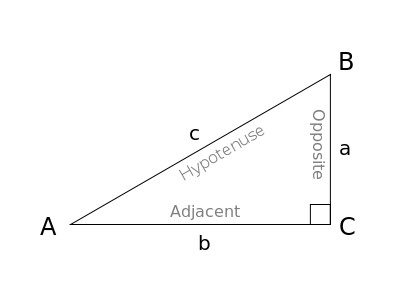### Sine

This is the simple equation that is used to work out a trigonometry question using Sine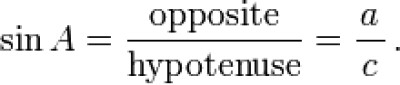This is the simple equation used to work out a trigonometry question using tangent.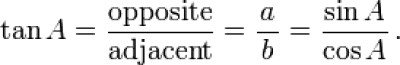# Algebra

## Plain sticky notes

### What does it mean when it says "Solve this Equation"?

If you are given a question like y+79≥13(½x-6) and are told to solve it, what that basically means is, make x the subject, or in other words, put x on one side of the ≥ sign with nothing else.

### BIG RULE!!!!!!

When making X the subject of an equation, you MUST do the same thing to both side of the = sign, for example if you had y=2x-4 and you were trying to get rid of the -4, you would add it to both sides, so that it cancels out on the side that with -4 and because you have to do the same thing to both sides.

# Simultaneous Equation

## Plain sticky notes

### Simultaneous Equations

You will first have to make two numbers the same, if you don't already have two, two do this you must multiply eith er the top or bottom equation, so that one of the numbers is the same as one of the numbers on the other equation, you may have to multiply both equations if so, do it the easiest way possible.

### Simultaneous Equations

If you are trying to work out simultaneous equations, you have to remember that if the two numbers you are trying to cancel out are the same in the way of if their positive or negative, you must minus them, if they are different in the way of positive or negative, you must add them.

### Simultaneous Equations

Click here to typOnce you have minused the bottom equation from the top one, you will have a simple equation which you must then solve by making the letter you have left the subject.

### Simultaneous Equations

After you have made that letter the subject you must substute that letter back into one of the original equations, I would personaly recomend putting it into the simplest equation, if so it would make you life that little bit easier. Once you've done this, you must then make the letter that you have left the subject of the equation. Once you have done this, you will have the value of the 2 letters.